RC Differentiator

Calculations & Observations

A differentiating circuit is a simple RC series circuit with output taken across the resistor R. The circuit is designed in such a way that output is proportional to the derivative of the input. Thus if a d.c. or constant input is applied to such a circuit, the output will be zero. It is because the derivative of the constant is zero.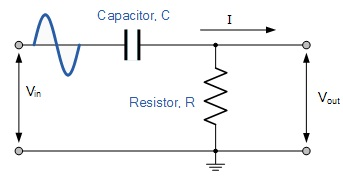Let Vi, be the input alternating voltage and let i be the resulting alternating current. The charge q on the capacitor at any instant is: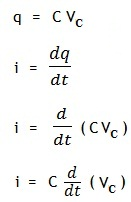Since the capacitive reactance is very larger than R, the input voltage can be consider equal to the capacitor voltage without any error, i.e. VC = Vi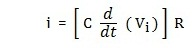Output voltage is given by: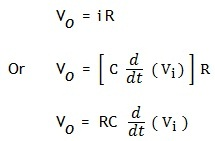Where RC is a constant, and
Hence,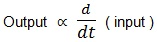The output waveform from a differentiating circuit depends upon the time constant and the shape of the input signal.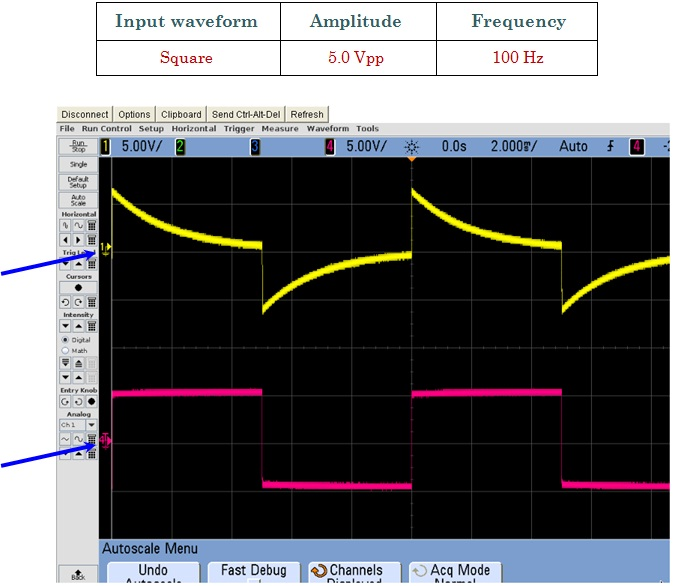Fig.1 Shows Input signal (Red wave form) and Output (Yellow Waveform)Fig.2 Shows Input signal (Red wave form) and Output (Yellow Waveform)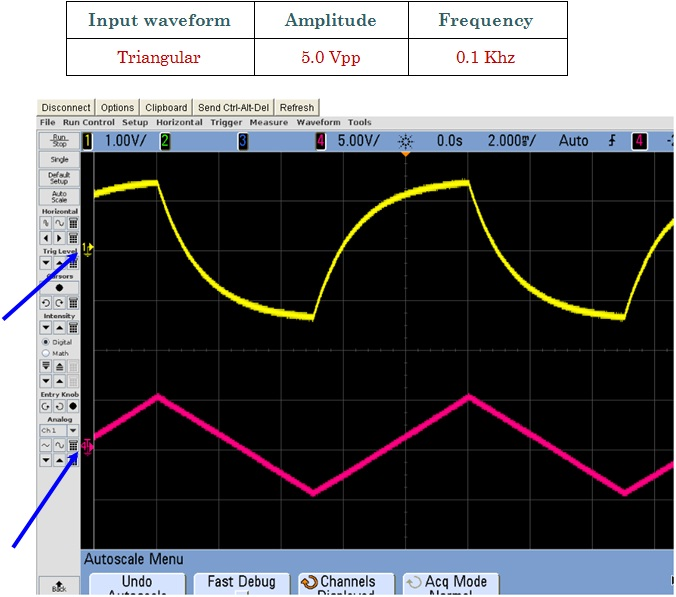Fig.3 Shows Input signal (Red wave form) and Output (Yellow Waveform)